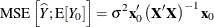### Mean Squared Error

The mean squared error is arguably the most important criterion used to evaluate the performance of a predictor or an estimator. (The subtle distinction between predictors and estimators is that random variables are predicted and constants are estimated.) The mean squared error is also useful to relay the concepts of bias, precision, and accuracy in statistical estimation. In order to examine a mean squared error, you need a target of estimation or prediction, and a predictor or estimator that is a function of the data. Suppose that the target, whether a constant or a random variable, is denoted as U. The mean squared error of the estimator or predictor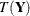for U is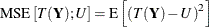The reason for using a squared difference to measure the loss betweenand U is mostly convenience; properties of squared differences involving random variables are more easily examined than, say, absolute differences. The reason for taking an expectation is to remove the randomness of the squared difference by averaging over the distribution of the data.

Consider first the case where the target U is a constant—say, the parameter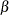—and denote the mean of the estimatoras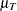. The mean squared error can then be decomposed as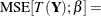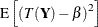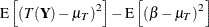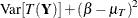The mean squared error thus comprises the variance of the estimator and the squared bias. The two components can be associated with an estimator’s precision (small variance) and its accuracy (small bias).

Ifis an unbiased estimator of—that is, if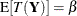—then the mean squared error is simply the variance of the estimator. By choosing an estimator that has minimum variance, you also choose an estimator that has minimum mean squared error among all unbiased estimators. However, as you can see from the previous expression, bias is also an average property; it is defined as an expectation. It is quite possible to find estimators in some statistical modeling problems that have smaller mean squared error than a minimum variance unbiased estimator; these are estimators that permit a certain amount of bias but improve on the variance. For example, in models where regressors are highly collinear, the ordinary least squares estimator continues to be unbiased. However, the presence of collinearity can induce poor precision and lead to an erratic estimator. Ridge regression stabilizes the regression estimates in this situation, and the coefficient estimates are somewhat biased, but the bias is more than offset by the gains in precision.

When the target U is a random variable, you need to carefully define what an unbiased prediction means. If the statistic and the target have the same expectation,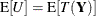, then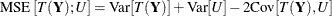In many instances the target U is a new observation that was not part of the analysis. If the data are uncorrelated, then it is reasonable to assume in that instance that the new observation is also not correlated with the data. The mean squared error then reduces to the sum of the two variances. For example, in a linear regression model where U is a new observation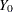andis the regression estimator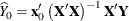with variance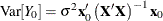, the mean squared prediction error foris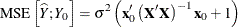and the mean squared prediction error for predicting the mean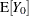is